### Home > CALC > Chapter 3 > Lesson 3.4.2 > Problem3-166

3-166.
1. Find the general antiderivative F(x) for each function below. Test your solution by verifying that F ′(x) = f(x). Homework Help ✎

1.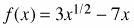2. f(x) = cos x + 2 sin x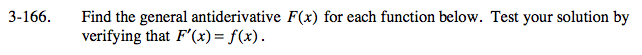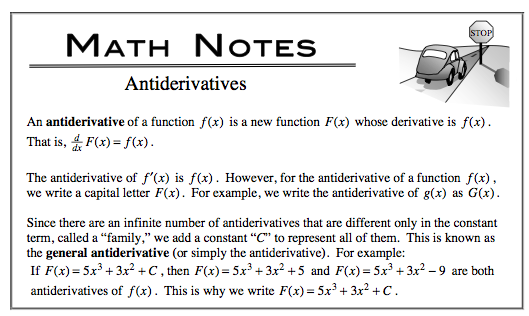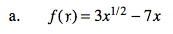Don't forget the vertical shift (+C).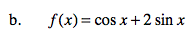Don't forget the vertical shift (+C).